# 旋转势阱耦合下孤子的研究Study on Solitons Coupled by Rotating Potential Well

DOI: 10.12677/PM.2021.114065, PDF, HTML, XML, 下载: 16  浏览: 66

Abstract: In this paper, we study the two-component Schrödinger equation. Under the cubic nonlinear self attraction, we introduce a special coupling action spiral potential well. The two components are coupled in spiral form. Through calculation, we get three stable mode solitons of the system, which are L soliton, M soliton and R soliton. We find that the mode of soliton can be switched by adjusting the rotation speed. At the same time, we also discuss the influence of rotational velocity and component coupling strength on the soliton wave function and soliton distribution.

1. 引言

2. 模型及理论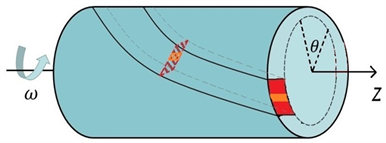Figure 1. Coupling cylindrical thin waveguide shell

$\begin{array}{l}i\frac{\partial }{\partial z}\psi =-\frac{1}{2}\frac{{\partial }^{2}}{\partial {\theta }^{2}}\psi +\gamma {|\psi |}^{2}\psi +{C}_{o}f\left(\theta -\omega z\right)\varphi \\ i\frac{\partial }{\partial z}\varphi =-\frac{1}{2}\frac{{\partial }^{2}}{\partial {\theta }^{2}}\varphi +\gamma {|\varphi |}^{2}\varphi +{C}_{o}f\left(\theta -\omega z\right)\psi \end{array}$ (1)

$\begin{array}{l}i\frac{\partial }{\partial z}\psi =-\frac{1}{2}\frac{{\partial }^{2}}{\partial {\theta }^{2}}\psi +i\omega \frac{\partial }{\partial \theta }\psi -{|\psi |}^{2}\psi -{C}_{o}f\left(\theta \right)\varphi \\ i\frac{\partial }{\partial z}\varphi =-\frac{1}{2}\frac{{\partial }^{2}}{\partial {\theta }^{2}}\varphi +i\omega \frac{\partial }{\partial \theta }\varphi -{|\varphi |}^{2}\varphi -{C}_{o}f\left(\theta \right)\psi \end{array}$ (2)

$i\frac{\partial }{\partial z}\chi \left(\theta ,z\right)=\stackrel{^}{L}\chi \left(\theta ,z\right)-{|\chi \left(\theta ,z\right)|}^{2}\chi \left(\theta ,z\right)$ (3)

$\chi \left(\theta ,z\right)=\left[\begin{array}{l}\psi \left(\theta ,z\right)\\ \varphi \left(\theta ,z\right)\end{array}\right]$$\stackrel{^}{L}=\left(\begin{array}{cc}\stackrel{^}{h}& -\stackrel{^}{C}\\ -\stackrel{^}{C}& \stackrel{^}{h}\end{array}\right)$

$\stackrel{^}{h}=-\frac{1}{2}\frac{{\partial }^{2}}{\partial {\theta }^{2}}+i\omega \frac{\partial }{\partial \theta }$$\stackrel{^}{C}={C}_{o}f\left( \theta \right)$

$\stackrel{^}{L}$ 为不含z变量的线性矩阵算符。

$\chi \left(\theta ,z\right)=\left[\begin{array}{l}\psi \left(\theta ,z\right)\\ \varphi \left(\theta ,z\right)\end{array}\right]=\left[\begin{array}{l}{\psi }_{s}\left(\theta \right)\\ {\varphi }_{s}\left(\theta \right)\end{array}\right]{\text{e}}^{iKz}$

$\chi \left(\theta ,z\right)=\left[\begin{array}{l}{\psi }_{s}\left(\theta \right)\\ {\varphi }_{s}\left(\theta \right)\end{array}\right]{\text{e}}^{iKz}={\chi }_{s}\left(\theta \right){\text{e}}^{iKz}$ (4)

$\stackrel{^}{L}{\chi }_{s}\left(\theta \right)-{|{\chi }_{s}\left(\theta \right)|}^{2}{\chi }_{s}\left(\theta \right)+K{\chi }_{s}\left(\theta \right)=0$

$\chi \left(\theta ,z\right)={\text{e}}^{iKz}\left[{\chi }_{s}\left(\theta \right)+\alpha \left(\theta \right){\text{e}}^{i\lambda z}+{\beta }^{\ast }\left(\theta \right){\text{e}}^{-i{\lambda }^{\ast }z}\right]$ (5)

$\left(\begin{array}{cc}-\stackrel{^}{L}+2{|{\chi }_{s}|}^{2}-K& {\chi }_{{}_{s}}^{2}\\ -{\left({\chi }_{{}_{s}}^{\ast }\right)}^{2}& {\stackrel{^}{L}}^{\ast }-2{|{\chi }_{s}|}^{2}+K\end{array}\right)\left(\begin{array}{l}\alpha \\ \beta \end{array}\right)=\lambda \left( \alpha \beta \right)$

(a) 分量中波函数的能量 ${P}_{\psi }$${P}_{\varphi }$，定义为

$\left\{{P}_{\psi },{P}_{\varphi }\right\}={\int }_{-\pi }^{\pi }\left\{{|\psi |}^{2},{|\varphi |}^{2}\right\}\text{d}\theta$

$P={P}_{\psi }+{P}_{\varphi }$

P为我们预设的系统(光场)的总能量。

(b) 集中分布在两个波导壳中的能量可能会出现差异，我们定义相对能量破缺率，

${\Theta }_{P}={\int }_{-\pi }^{\pi }|{|\psi |}^{2}-{|\varphi |}^{2}|\text{d}\theta /P$

(c) 波形的对称性可以通过以下定义判别，

${\Theta }_{\chi }={\int }_{0}^{\pi }|{|\chi \left(\theta \right)|}^{2}-{|\chi \left(-\theta \right)|}^{2}|\text{d}\theta /{P}_{\chi }$

${\Theta }_{\chi }$ 等于0，则波形 ${|\chi |}^{\text{2}}$ 具有对称性。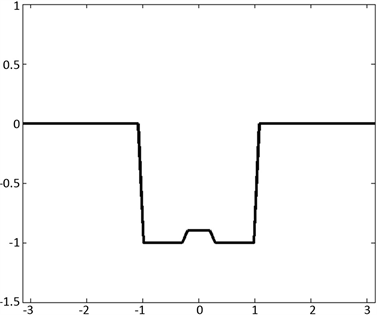Figure 2. Potential well function

3. 数值结果与分析

3.1. 稳定模式解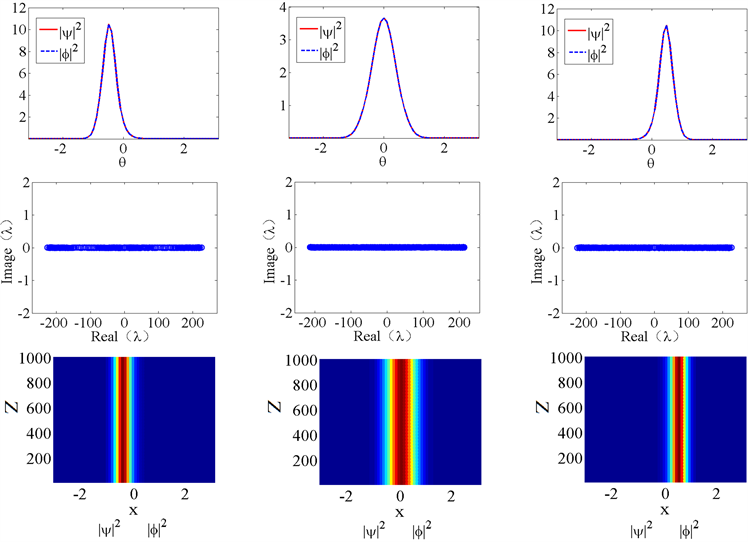Figure 3. Three modes of soliton solution ( ${\Theta }_{P}=0$ )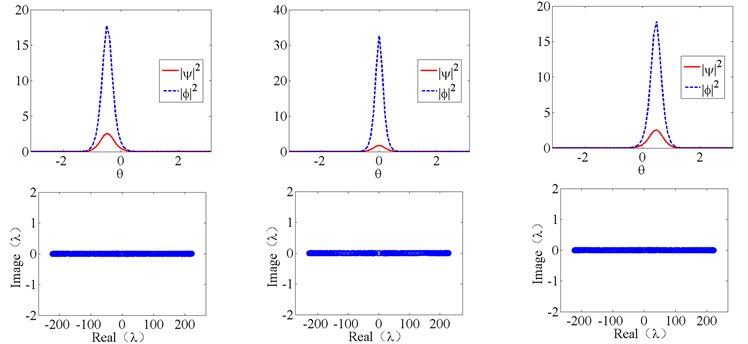Figure 4. Three modes of soliton solutions ( ${\Theta }_{P}>0$ )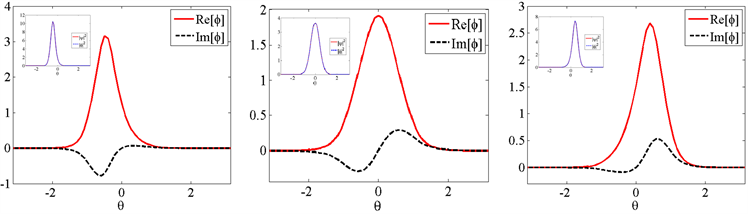Figure 5. The real and imaginary part of the wave function graph

$\varphi =|\varphi |{\text{e}}^{i\phi },\phi =\mathrm{arctan}\left(\frac{\mathrm{Im}\left[\varphi \right]}{\mathrm{Re}\left[\varphi \right]}\right)$ (6)

3.2. 非稳定模式解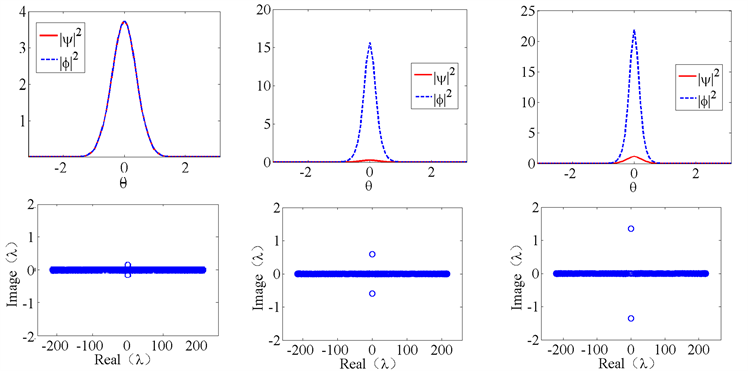Figure 6. Pseudo M soliton

3.3. 旋转速度的调控作用

3.4. 耦合作用的调控作用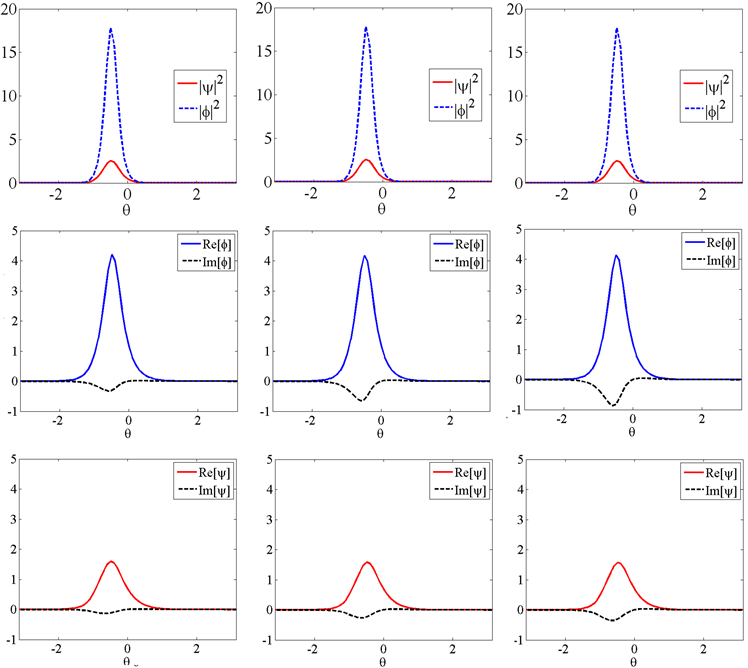(a) L孤子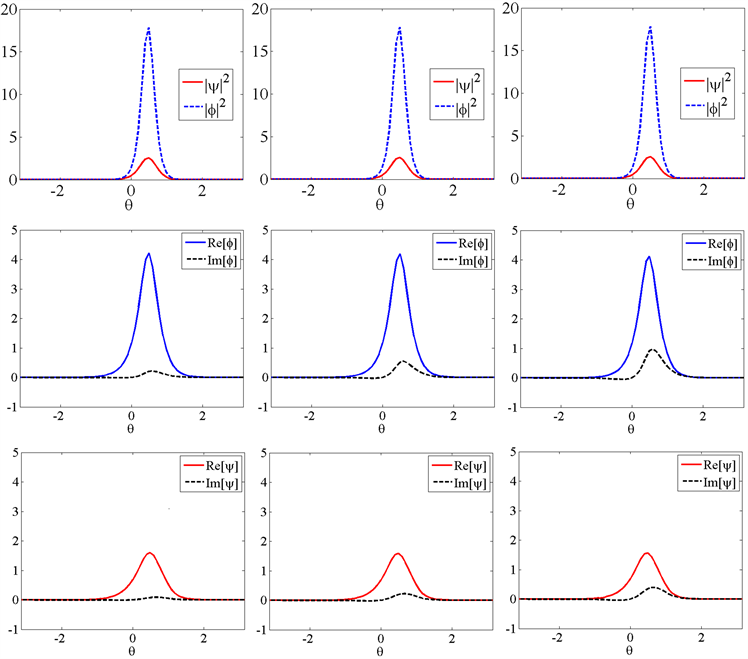(b) R孤子

Figure 7. Waveforms of soliton at different rotation speeds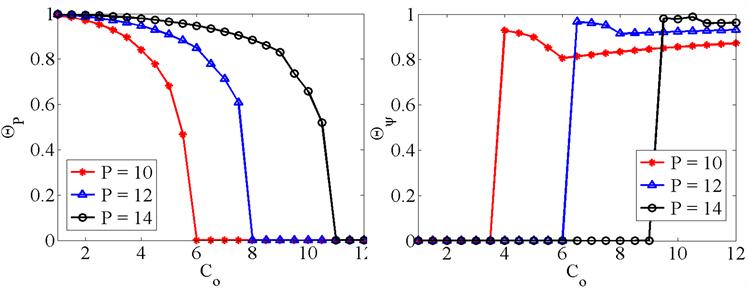(a) (b)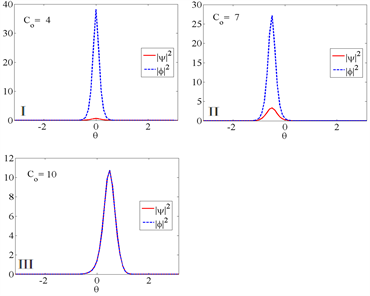(c) (d)

Figure 8. The effect of coupling on soliton

4. 结论

  Korteweg, D.J. and De Vries, G. (2011) On the Change of Form of Long Waves Advancing in a Rectangular Canal, and on a New Type of Long Stationary Waves. Philosophical Magazine, 91, 1007-1028. https://doi.org/10.1080/14786435.2010.547337  Khaykovich, L., Schreck, F., Ferrari, G., Bourdel, T., Cubizolles, J., Carr, L.D., Castin, Y. and Salomon, C. (2002) Formation of a Matter-Wave Bright Soliton. Science, 296, 1290-1293. https://doi.org/10.1126/science.1071021  Chiao, R.Y. and Wu, Y.S. (1986) Manifestations of Berry’s Topological Phase for the Photon. Physical Review Letters, 57, 933-936. https://doi.org/10.1103/PhysRevLett.57.933  Tomita, A. and Chiao, R.Y. (1986) Observation of Berry’s Topological Phase by Use of an Optical Fiber. Physical Review Letters, 59, 937-940. https://doi.org/10.1103/PhysRevLett.57.937  Ornigotti, M., Valle, G.D., Gatti, D., et al. (2007) Topological Suppression of Optical Tunneling in a Twisted Annular Fiber. Physical Review A, 76, Article ID: 023833. https://doi.org/10.1103/PhysRevA.76.023833  Kanamoto, R., Carr, L.D. and Ueda, M. (2008) Topological Winding and Unwinding in Metastable Bose-Einstein Condensates. Physical Review Letters, 100, Article ID: 060401. https://doi.org/10.1103/PhysRevLett.100.060401  Guo, H., Chen, Z., Liu, J., et al. (2014) Fundamental Modes in a Waveguide Pipe Twisted by Inverted Nonlinear Double-Well Potential. Laser Physics, 24, Article ID: 045403. https://doi.org/10.1088/1054-660X/24/4/045403  Li, Y.Y., Pang, W. and Malomed, B.A. (2014) Wave Modes Trapped in Rotating Nonlinear Potentials. In: Localized Excitations in Nonlinear Complex Systems, Springer International Publishing, Berlin, 171-192. https://doi.org/10.1007/978-3-319-02057-0_8  Li, Y.Y., Pang, W. and Malomed, B.A. (2012) Nonlinear Modes and Symmetry Breaking in Rotating Double-Well Potentials. Physical Review A, 86, 94-101. https://doi.org/10.1103/PhysRevA.86.023832  Chen, G.H., Wang, H.C., Chen, Z.P., et al. (2016) Fundamental Modes in Waveguide Pipe Twisted by Saturated Double-Well Potential. Frontiers of Physics, 12, Article ID: 124201. https://doi.org/10.1007/s11467-016-0601-6  Luo, Z.H., Li, Y.Y., Pang, W., Liu, Y. and Wang, X.J. (2013) Double Symmetry Breaking of Modes in Dual-Core Rotating System. Journal of the Physical Society of Japan, 82, Article ID: 124401. https://doi.org/10.7566/JPSJ.82.124401  Luo, W., Chen, Z., Zou, Z., Luo, Z., et al. (2019) Symmetry and Asymmetry Nonlinear Modes in Dual Cylinder Waveguide Shells Coupled by a Rotating Double-Well Connection. Journal of Nonlinear Optical Physics & Materials, 28, Article ID: 1850039. https://doi.org/10.1142/S021886351850039X